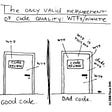# Handling Floating-Point Numbers and BigDecimal class in JAVA

Computers use a fixed number of significant digits to represent a floating-point number and this is scaled using an exponent in some fixed base. There are many floating-point representations available. Through this article let's look in detail at the most commonly used standard. This is called IEEE 754 Standard for Floating-Point Arithmetic. This representation is used in most of the intel based PCs, Macs, and Unix platforms.

There are 3 main components in the IEEE 754 Standard.

1. Signed Bit/ Sign of Mantissa — Used the represent the sign of the value. 0 for a positive number and 1 for a negative number.
2. Biased Exponent — This field needs to represent both positive and negative exponents. The final exponent is calculated by the addition of exponent bias to the existing exponent.
3. Mantissa — Used to represent the precision bits of the number.

These can be divided into 3 main categories based on the above components.

# Conversion of a Floating number to Binary ( IEEE 754 Standard )

Example: Consider the Number 9.1.

Step 01: Divide the number into 2 parts as an integral part and a decimal part.

Step 02: Calculate the equivalent binary numbers to these 2 parts separately.

Step 03: Combine these 3 numbers and get the result in the scientific format.

Step 04: Now we need to write this in the IEEE 754 Standard(Single).

• Signed Bit — 0 because the number is positive.
• Exponent — As mentioned in the first section, the exponent should be able to hold both positive and negative values. Therefore we need to add the exponent bias.

Exponent — 3 + 127 = 130

Exponent in Binary = 10000010 (8 bits long)

• Mantissa — In order to take the mantissa, we have to get the calculated decimal part up to 23 bits (Size of Mantissa).

Step 05: Combine the values and get the final result.

However, The answer will be different if we use a calculator or an electronic device to do this calculation(with IEEE 754 standard). That is due to the Floating point rounding problem.

01000001000100011001100110011010

# Floating Point Rounding Problem

Consider the above scenario. The answer generated by the computer is different. What's the reason for this change?

The size of the mantissa is 23 bits. However, the answer we got for the above value exceeds 23 bits. In such scenarios, the computer will perform the following steps.

If the 24th bit is 0 — Remove all values from the 24th bit and get the number.

If the 24th bit is 1 — Add 1 to the 23rd bit and remove the rest of the values from the 24th bit.

In the above scenario, the 24th bit is 1. Therefore 1 is getting added to the 23rd bit. And due to that, the value is different. This is called the floating-point rounding problem.

This happens due to the use of incorrect data types. As an example, the use of double data type to hold currencies is problematic.

Now let’s focus on a practical problem. Consider the following scenario.

Output —

The result of this loop will be an infinite loop. So that is because the value of “i” will never be 0. An additional value is getting added to the end. So it will never be 0.

# BIG Decimal to the rescue

We can use Big decimal class to overcome this problem. This class provides various operations of double numbers. Arithmetic operations, Scale handling, Comparison, format conversions are some of them.

This class can easily handle both small and large floating-point numbers with great precision. Big decimal class is available in java.math package. We can easily create an Object from Big decimal class and perform necessary operations. Consider the following example.

As depicted in the above segment, Big decimal class provides various methods which can be used to perform these necessary operations. The article under the reference section will explain more about all the available methods and constructors in the Big Decimal class.

# References —

--

--

--

## More from Damsak Bandara

Associate Software Engineer at Virtusa

Love podcasts or audiobooks? Learn on the go with our new app.

## Symfony Internals #1: Inside the Framework Configuration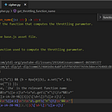## How to access and secure the Docker Engine API in Docker Swarm for AWS## How To Host Flutter Web In Linux Using Nginx## The casino technique for generating random numbers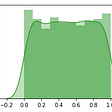## Atomic Operation In C#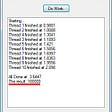## PROVISIONING EMR CLUSTER USING TERRAFORM## Damsak Bandara

Associate Software Engineer at Virtusa

## Resume Parsing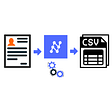## Keeping it Clean — the Art of Clean Coding